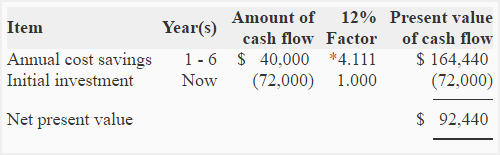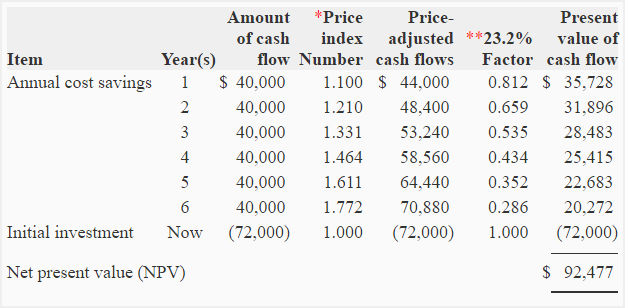# Problem-6 (Capital budgeting/NPV with inflation)

Delta company manufactures silicon boards that are used in preparing small, medium and large size electronic circuits. The company is considering to reduce its cost by automating some of its manufacturing tasks. This automation requires the installation of a new equipment. The relevant information for net present value (NPV) analysis of investment in new equipment is given below:

• Cost of equipment: \$72,000
• Expected annual cost savings to be provided by new equipment: \$40,000
• Useful life of the equipment: 6 years
• Salvage value at the end of 6 years: \$0
• Cost of capital: 23.2%
• Expected inflation rate in cash flows associated with the new equipment: 10%

Required:

1. What would be the net present value (NPV) of new equipment if:
(a). the inflation is considered?
(b). the inflation is not considered?
2. Should the new equipment be purchased?

## Solution:

### (1) Computation of net present value:

a. If inflation is not considered:

In this problem, we are given the nominal discount rate of 23.2%. In order to compute NPV without considering inflation, the first step is to compute the real discount rate. It can be computed by using the following formula:

Real discount rate = (Nominal discount rate – Inflation rate) ÷ (1 + Inflation rate)

= (23.2% – 10%) ÷ (1 + 10%)

= (13.2%) ÷ 1.1

= 12%

The real discount rate is 12% and has been used in the following computations.* Value from “present value of an annuity of \$1 in arrears table“.

b. If inflation is considered:*(1 + inflation rate)n
**1/(1 + i)n

The net present value computed with and without inflation should be the same. The difference of \$37 (\$92477 – \$92,440) in NPV figure computed under two approaches is due to rounding error.

### (2) Conclusion:

The positive net present value indicates that the project is acceptable. The new equipment should, therefore, be purchased to automate some manufacturing process.

A D V E R T I S E M E N T
3 Comments on Problem-6 (Capital budgeting/NPV with inflation)
1.Marina

without formula is very difficult to understand how you got the numbers….

2.ebere glory

3.